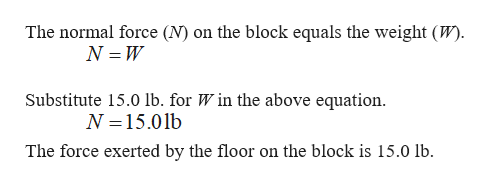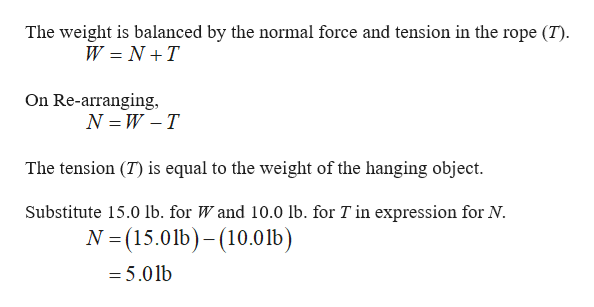# A 15.0-lb block rests on a horizontal floor. (a) Whatforce does the floor exert on the block? (b) A ropeis tied to the block and is run vertically over a pulley.The other end is attached to a free-hanging 10.0-lbobject. What now is the force exerted by the floor on the15.0-lb block? (c) If the 10.0-lb object in part (b) is replacedwith a 20.0-lb object, what is the force exerted by the floor onthe 15.0-lb block?

Question
1 views

A 15.0-lb block rests on a horizontal floor. (a) What
force does the floor exert on the block? (b) A rope
is tied to the block and is run vertically over a pulley.
The other end is attached to a free-hanging 10.0-lb
object. What now is the force exerted by the floor on the
15.0-lb block? (c) If the 10.0-lb object in part (b) is replaced
with a 20.0-lb object, what is the force exerted by the floor on
the 15.0-lb block?

check_circle

Step 1

The force exerted by the floor on the block ishelp_outlineImage TranscriptioncloseThe normal force (N) on the block equals the weight (W). N = W Substitute 15.0 lb. for W in the above equation. N =15.01b The force exerted by the floor on the block is 15.0 lb. fullscreen
Step 2

The force exerted by the floor on the block wh...help_outlineImage TranscriptioncloseThe weight is balanced by the normal force and tension in the rope (T). W = N +T On Re-arranging, N = W -T The tension (T) is equal to the weight of the hanging object. Substitute 15.0 lb. for W and 10.0 lb. for T in expression for N. N =(15.01b)– (10.01b) = 5.01b fullscreen

### Want to see the full answer?

See Solution

#### Want to see this answer and more?

Solutions are written by subject experts who are available 24/7. Questions are typically answered within 1 hour.*

See Solution
*Response times may vary by subject and question.
Tagged in

### Newtons Laws of Motion